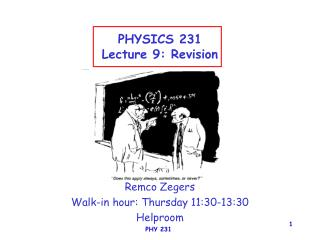# PHYSICS 231 Lecture 9: Revision - PowerPoint PPT PresentationDownload PresentationPHYSICS 231 Lecture 9: Revision

PHYSICS 231 Lecture 9: Revision
Download Presentation## PHYSICS 231 Lecture 9: Revision

- - - - - - - - - - - - - - - - - - - - - - - - - - - E N D - - - - - - - - - - - - - - - - - - - - - - - - - - -
##### Presentation Transcript

1. PHYSICS 231Lecture 9: Revision Remco Zegers Walk-in hour: Thursday 11:30-13:30 Helproom PHY 231

3. Units • Convert all units in your problem to be in the SI system • When adding/subtracting two quantities check whether their units are the same. • If you are unsure about an equation that you want to use, perform the dimensional analysis and make sure that each part of the equation that is set equal/subtracted/added have the same dimensions • When 2 quantities are multiplied, their units do not have to be the same. The result will have as unit the multiplied units of the quantities being multiplied. • Sin, cos and tan of angles are dimensionless PHY 231

4. Solving a quadratic equation At2+Bt+c=0 • Use the equation: • CHECK THE ANSWER • OR just calculate it! • t2+(B/A)t+C/A=0 and use (t+d)2=t2+2dt+d2 • [t+(B/2A)]2-B2/4A2+C/A=0 • t=-B/2A(B2/4A2-C/A) PHY 231

5. Constant velocity Constant acceleration Constant motion x(t)=x0+v0t+½at2 x(t)=x0 x(t)=x0+v0t x(m) x(m) x(m) t t t v m/s v m/s v m/s v(t)=0 v(t)=v0+at v(t)=v0 t t t a m/s2 a m/s2 a m/s2 a(t)=0 a(t)=0 a(t)=a0 t t t PHY 231

6. x v t time PHY 231

7. A+B Vector operations in equations (xa+b,ya+b) y (xb,yb) B (xa,ya) A x Xa=Acos() Ya=Asin() length/magnitude of A: (Xa2+Ya2) PHY 231

8. vx=v0cos vy=v0sin-2g=0 vx=v0cos vy=v0sin-1g vx=v0cos vy=v0sin-3g V=v0 vx=v0cos vy=v0sin vx=v0cos vy=v0sin-4g  vx=v0cos vy=v0sin-5g Parabolic motion: decompose x and y directions t=0 t=1 t=2 t=3 t=4 t=5 PHY 231

9. X=X0 Y=Y0  Parabolic motion X(t)=X0+V0cost Y(t)=Y0+V0sint-1/2gt2 t=0 t=1 t=2 t=3 t=4 t=5 PHY 231

10. 2D motion • When trying to understand the motion of an object in 2D decompose the motion into vertical and horizontal components. • Be sure of your coordinate system; is the motion of the object you want to study relative to another object? • Write down the equations of motion for each direction separately. • If you cannot understand the problem, draw motion diagrams for each of the directions separately. • Make sure you understand which quantity is unknown, and plug in the equation of motions the quantities that you know (givens). Then solve the equations. PHY 231

11. Newton’s Laws • First Law: If the net force exerted on an object is zero the object continues in its original state of motion; if it was at rest, it remains at rest. If it was moving with a certain velocity, it will keep on moving with the same velocity. • Second Law: The acceleration of an object is proportional to the net force acting on it, and inversely proportional to its mass: F=ma • If two objects interact, the force exerted by the first object on the second is equal but opposite in direction to the force exerted by the second object on the first: F12=-F21 PHY 231

12. General strategy for problems with forces • If not given, make a drawing of the problem. • Put all the relevant forces in the drawing, object by object. • Think about the axis • Think about the signs • Decompose the forces in direction parallel to the motion and perpendicular to it. • Write down Newton’s first law for forces in the parallel direction and perpendicular direction. • Solve for the unknowns. • Check whether your answer makes sense. PHY 231

13. horizontal Vertical h x y vx t t Vy X(t)=x0+voxt =0+12.7t =34 (hit the ground) t=34/12.7=2.68 s Y(t)=y0+v0yt-0.5gt2 =h-0.5gt2 =0 (hits the ground) so: h=0.5gt2 h=35 m A snowball is launched horizontally from the top of a building at v=12.7 m/s. If it lands 34 m away from the bottom, how high was the building? V0=12.7 m/s h? d=34m PHY 231

14. blue Blue ball: yb(t)=y0+v0bt-0.5gt2= =13.3-5.t-0.5gt2 red 13.3 v0r x x Red ball: yr(t)=yo+vort-0.5gt2= =26.8t-0.5gt2 t t v0b v v yb(t)=yr(t) 13.3-5t-0.5gt2=26.8t-0.5gt2 13.3=31.8t so t=0.42s A red ball is thrown upward with a velocity of 26.8 m/s. A blue ball is dropped from a 13.3 m high building with initial downward velocity of 5.00 m/s. At what time will the balls be at the same height. Vo=-5.00 h=13.3m V0=26.8 PHY 231

15. v t 5.05+ 2.96 5.09 In first period: v(t)=v0+at=0+1.48·5.09=7.53 m/s In 2nd period: v(t)=v0+at=7.53-1.91·2.96=1.88 m/s A car starts at rest and travels for 5.09 s with uniform acceleration of +1.48 m/s2. The driver then brakes, causing uniform acceleration of -1.91 m/s2. If the brakes are applied for 2.96 s how fast is the car going after that? PHY 231

16. Horizontal Vertical Due to tide: 3000 N 0 N Due to wind: 6000cos(135)=-4243 6000sin(135)=+4243 Sum: -1243 N 4243 N N 6000N Magnitude of resulting force: Fsum=[(-1243)2+(4243)2]=4421 N Direction: angle=tan-1(4243/-1243)= 1060 (calc: -730, add 1800) F=ma so a=F/m=4421/2000=2.21 m/s2 W E 3000N S A 2000 kg sailboat is pushed by the tide of the sea with a force of 3000 N to the East. Because of the wind in its sail it feels a force of 6000 N toward to North-West direction. What is the magnitude and direction of the acceleration? PHY 231

17. TVerL horizontal Vertical left rope Tsin(45) Tcos(45) right rope -Tsin(45) Tcos(45) gravity 0 -1*9.81 Sum: 0 2Tcos(45)-9.81 TVerR 450 450 ThorL Object is stationary, so: 2Tcos(45)-9.81=0 so, T=6.9 N Fg T T 900 1kg A mass of 1 kg is hanging from a rope as shown in the figure. If the angle between the 2 supporting wires is 90 degrees, what is the tension in each rope? ThorR PHY 231